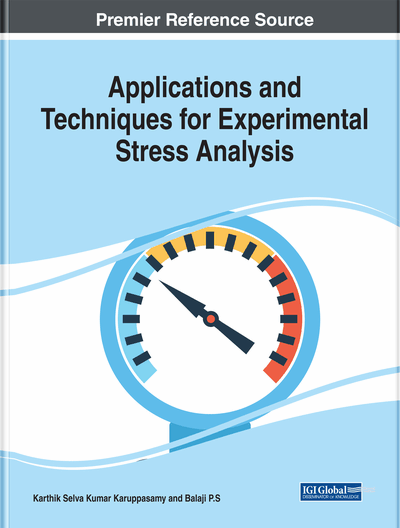# Modelling Stress Distribution in a Flexible Beam Using Bond Graph Approach

Jay Prakash Tripathi (Thapar Institute of Engineering and Technology, India)
DOI: 10.4018/978-1-7998-1690-4.ch011

## Abstract

The bond graph (BG) approach of modelling provides a unified approach for modelling the systems having components belonging to multi-energy domains. Moreover, as evident by its name, it is a graphical approach. The graphical nature provides a tool for conceptual visualization of the model. It also provides some algorithmic tools because of its formal structure and syntax, thereby enabling model consistency checks such as checking algebraic loops, etc. There are a large number of texts published in recent years that may be refereed for background material on the BG methodology. Though used in many applications, its use in modelling stress distribution in the system is limited. Finite element (FE) modelling has found wide applicability for the same. This chapter is aimed at providing background knowledge, a comparison of BG approach with the FE approach, and a review of research progress of past two decades in this direction.
Chapter Preview
Top

## Introduction

Interdisciplinary research is common, with its broad or complex problems needing to be solved by a single discipline. Conceptual unification must shrink the many interdisciplinary techniques into one common method. One example of conceptual unification, the bond graph (BG) technique, includes the modeling, simulation, control, and synthesis of dynamics of physical systems with different energy domains. Paynter (1961) invented the BG technique at the Massachusetts Institute of Technology. Apart from conceptual unification, the BG also provides the following benefits:

• Modular Approach of Modeling: Each component can be modeled into individual blocks for easy conversion, correction, and reuse.

• Dynamic Model from Kinematic Constraints: Using the BG algorithm, a fully dynamic model and equations of motion are directly obtained from kinematic constraints and energy balance.

• Graphical Approach of Modeling andAnalysis: BG modeling is done in a well-defined, systematic order. This provides a structure and causality within the model for qualitative information.

The BG approach provides a unified approach for modeling systems with components belonging to multienergy domains. Moreover, as evident by its name, it is a graphical approach. Its graphical nature provides a tool for conceptual visualization of the model. It also provides algorithmic tools due to its formal structure and syntax, enabling a model consistency check (for example, checking algebraic loops).

There is a large number of texts published in recent years that may be refereed for background material on the BG methodology. Though used in many applications, there is limited information on its use in modeling stress distribution. However, finite element (FE) modeling has found wide applicability.

The objective of this chapter is to achieve two goals. First, it reviews recent advancements in the field of modeling stress distribution using the BG technique. Second, it is concise, attempting to incorporate all information required to understand the basics of modeling stress distribution using the BG technique.

Top

## Basics Of Bg Modeling

Power is a common variable exchanged between domains. It provides a basis for unification.Variables of energy domains may be generalized based on two factors of power: (1) effort (e); and (2) generalized flow (f). The effort and flow variables in mechanical domains are given in Table 1.

Table 1.
Effort (e) and flow (f) variables in mechanical domains
 Effort (e) Flow (f) Force(F) Velocity(v) Torque(τ) or moment Angular velocity (ω) Pressure (P) Volume flow rate (Q) Temperature (T) Entropy change rate (Ṡ) Stress (σ) Strain rate ()

## Complete Chapter List

Search this Book:
Reset